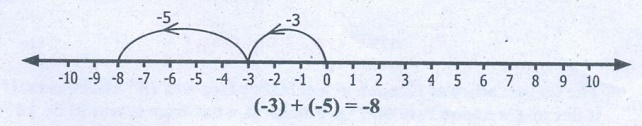Home | | Maths 7th Std | Exercise 1.1 (Properties of Addition)

# Exercise 1.1 (Properties of Addition)

7th Maths : Term 1 Unit 1 : Number System : Exercise 1.1 : Fill in the blanks, Say true or false, Objective type questions, Text Book Back Exercises Questions with Answers, Solution

Exercise 1.1

1. Fill in the blanks

(i) (−30) + 90 = 60

(ii) (−5) + –95 = −100

(iii) (−52) + (−52) = –104

(iv) 22 + (−22) = 0

(v) 140 + (−70) = 70

(vi) 20+80+ (–100) =0

(vii) 75 + (−25) = 50

(viii) 171+ (–171) = 0

(ix) [(−3)+ (−12)] + (−77) = ( –3) + [(−12)+ (−77)]

(x) (−42)+ [ 15 + (−23)] = [–42 +15] + –23.

2. Say true or false.

(i) The additive inverse of (–32) is (–32) (False)

(ii) (−90)+( −30) = 60 (False)

(iii) (−125)+25 = −100 (True)

(i) 8 and –12 using number line8 + 12 = –4

(ii) (–3) and (–5) using number line(–3) +(–5) = –8

(iii) (−100)+ (−10) = –110

(iv) 20 + (−72) = –52

(v) 82 + (−75) = 7

(vi) −48 + (−15) = –63

(vii) −225 + (−63) = –288

4. Thenmalar appeared for competitive exam which has negative scoring of 1 mark for each incorrect answer. In paper I she answered 25 questions incorrectly and in paper II, 13 questions incorrectly. Find the total reduction of marks.

Total reduction of marks = (–25) + (–13)

= –38

5. In a quiz competition, Team A scored +30,–20, 0 and team B scored –20, 0, +30 in three successive rounds. Which team will win? Can we say that we can add integers in any order?

TeamAscored = +30 – 20 + 0 = +10

Team B scored = –20 + 0+ 30 = +10

Team A = Team B

Yes, it can be added in any order.

6. Are (11 + 7) +10 and 11 + (7 +10) equal? Mention the property.

(11+7) + 10 = 18 + 10 = 28

11 + (7+10) = 11 + 17 = 28

(11+7) + 10 = 11 + (7+10)

Both are equal

7. Find 5 pairs of integers that add up to 2.

0 + 2, 1 + 1=2, –1+3 = 2, –2 + 4 = 2, –3 + 5 = 2

Objective type questions

8. The temperature at 12 noon at a certain place was 18 º at above zero. If it decreases at the rate of 3 º per hour at what time it would be 12 º below zero?

(i) 12 mid night

(ii) 12 noon

(iii) 10 am

(iv) 10 pm

9. Identify the problem with negative numbers as its answer:

(i) −9+(−5)+6

(ii) 8+(−12)−6

(iii) −4+2+10

(iv) 10+(–4)+8

Answer : –9 – (–5) + 6

10. (−10)+ (+7) = ____

(i) +3

(ii) –3

(iii) −17

(iv) +17

11. (−8)+10 + (−2) = ____

(i) 2

(ii) 8

(iii) 0

(iv) 20

12. 20 + (−9)+ 9 = ____

(i) 20

(ii) 29

(iii) 11

(iv) 38

Exercise 1.1

1. (i) 90 (ii) −95 (iii) −104 (iv) 22 (v)140 (vi) −100 (vii) 50 (viii) –171 (ix) −3 (x) 15, −42 , −23

2. (i) False (ii) False (iii) True

3. (i) −4 (ii) −8 (iii) −110 (iv) –52 (v)7 (vi) −63 (vii) −288

4. –38

5. Team A = Team B (yes, it can be added in any order)

6. Both are equal. Associative property under Addition

7. 0 + 2 , 1 + 1 = 2 , −1 + 3 , −2 + 4 , −3 + 5 (any pair)

Objective type questions

8. (iv) 10 pm

9. (i) –9+(–5)+6

10.(ii) –3

11. (iii) 0

12. (i) 20

Tags : Questions with Answers, Solution | Number System | Term 1 Chapter 1 | 7th Maths , 7th Maths : Term 1 Unit 1 : Number System
Study Material, Lecturing Notes, Assignment, Reference, Wiki description explanation, brief detail
7th Maths : Term 1 Unit 1 : Number System : Exercise 1.1 (Properties of Addition) | Questions with Answers, Solution | Number System | Term 1 Chapter 1 | 7th Maths